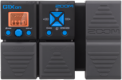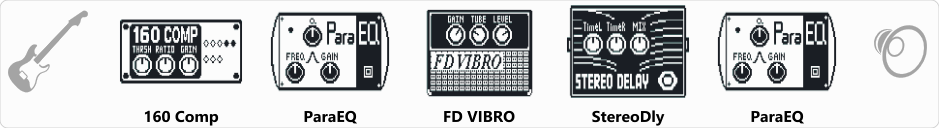Discussion in 'Zoom G1on/G1Xon' started by gilou5469, Jun 10, 2021.Device: Zoom G1Xon
Firmware: 1.21

Optimized for: Guitar Amp

Effects chain:Effect: "160 Comp" (Dynamics / Filter), active - "yes"
"THRSH" = -32
"Ratio" = 3.6
"Gain" = 0
"Knee" = Hard
"Level" = 103

Effect: "ParaEQ" (Dynamics / Filter), active - "yes"
"Freq1" = 250Hz
"Q1" = 1
"Gain1" = 3
"Freq2" = 3.2kHz
"Q2" = 1
"Gain2" = 11
"Level" = 76

Effect: "FD VIBRO" (Amp simulators), active - "yes"
"Gain" = 21
"Tube" = 27
"Level" = 142
"Trebl" = 17
"Middl" = 67
"Bass" = 58
"Prese" = 0
"CAB" = ALIEN 4x12
"OUT" = LINE

Effect: "StereoDly" (Delay / Reverb), active - "yes"
"TimeL" = 126
"TimeR" = 441
"Mix" = 64
"LchFB" = 24
"RchFB" = 54
"Level" = 114
"LchLv" = 75
"RchLv" = 100
"Tail" = Off

Effect: "ParaEQ" (Dynamics / Filter), active - "yes"
"Freq1" = 250Hz
"Q1" = 0.5
"Gain1" = 3
"Freq2" = 2.5kHz
"Q2" = 1
"Gain2" = 4
"Level" = 90

Patch Volume: 100

Note: This is a patch file, you will need to download and install the ToneLib-Zoom software to use the patch.